Next Previous Up Contents
Up: Plot Windows
Previous: Sphere Axes Control

### A.4.13 Corner Plot Window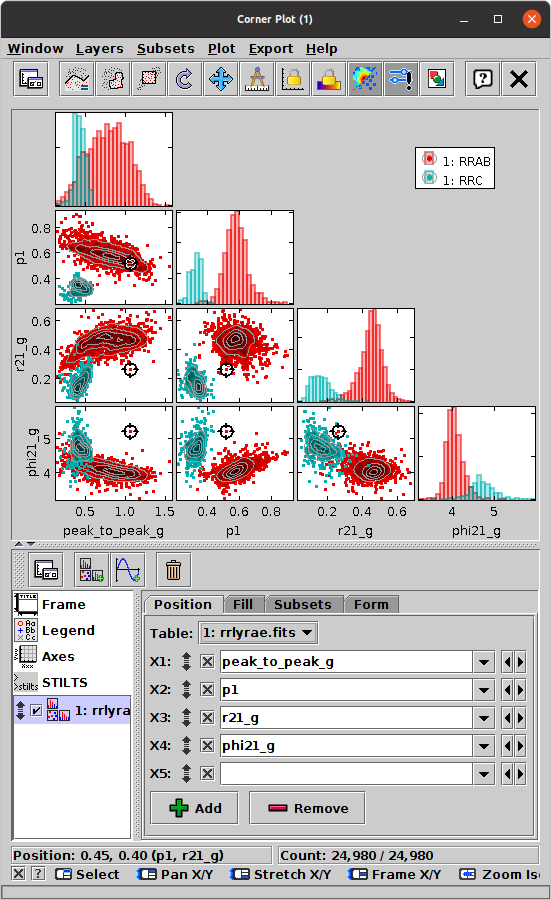Corner Plot Window

The Corner Plot (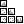) represents the relationships between multiple quantities by drawing a scatter-like plot of every pair of coordinates, and/or a histogram-like plot of every single coordinate, and placing these on (half or all of) a square grid. The horizontal coordinates of all the plots on each column, and the vertical coordinates of all the plots on each row, are aligned. The plots are all linked, so you can make graphical selections or click on a point to activate it in one of the panels, and the other panels will immediately reflect that action. Single-coordinate (histogram-like) plots appear on the diagonal, and coordinate-pair (scatter plot-like) plots appear off diagonal. By default only the diagonal and sub-diagonal part of the resulting plot matrix is shown, since the plots above the diagonal are equivalent to those below it, but this is configurable. This representation is variously known as a corner plot, scatter plot matrix, splom or pairs plot.

In principle any number of quantities can be simultaneously compared in this way, but attempting to use too many will make the individual plots too small to be useful. Depending on the size of your screen, you may find about 20 is a reasonable upper limit, though more detail will be visible with fewer. Note that the Float Controls (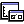) button (or equivalent action in the Window menu) can be used to detach the control panel from the plot display and so free up more space for the visualisation itself.

By default the linear size of the plot grid will be defined by the highest-numbered non-blank coordinate that is visible in any of the active Position layer controls, but it can be adjusted directly in the Axes control (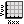).

The configuration of the plots is done in the same way as for the Plane Plot, and many of the plot types that are available there can be used in the grid cells of this plot. The Corner Plot currently offers only one plot control type:

•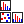Matrix Layer Control, containing these scatter-plot like actions:
•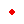Mark
•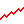Line
•Linear Fit
•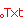Label
•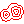Contour
•Grid
•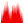Fill
•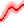Quantile
and these histogram-like actions:
•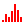Histogram
•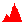KDE
•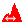KNN
•Densogram
•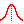Gaussian
The scatter-plot-like options will generate plots in the off-diagonal positions of the grid, and the histogram-like ones will generate plots on the leading diagonal. By default the Mark and Histogram options are both selected, but you can hide these as well as adding others.

As well as the standard actions, this window additionally provides the following toolbar buttons:

See the Window Overview for features common to all plotting windows. The following subsections describe navigation and axis configuration.

Note: the Corner Plot Window is new at TOPCAT version 4.9. Some of the features are experimental and may be changed in future releases.

Next Previous Up Contents